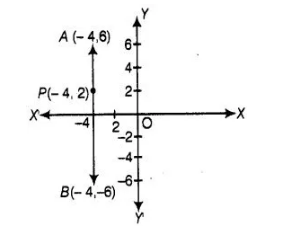# The point P(- 4, 2) lies on the line

Question:

The point P(- 4, 2) lies on the line segment joining the points A(- 4, 6) and B(- 4, – 6).

Solution:

True

We plot all the points P(-4,2),  A(-4, 6) and B(-4, – 6) on the graph paper,From the figure, point P(- 4,2) lies on the line segment joining the points A(- 4,6) and B(- 4, – 6),